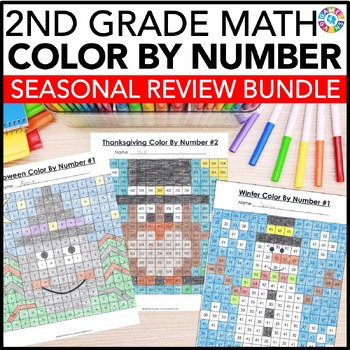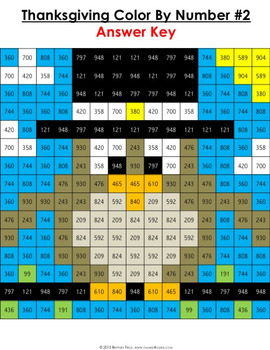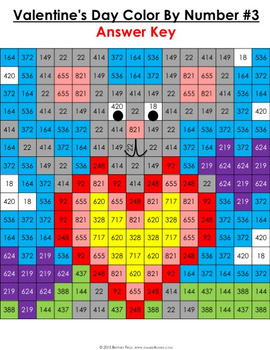# 2nd Grade Math Review Worksheets: Holidays Color by Number Math BundleResource Type
Format
Zip (167 MB|250+)
\$36.40
Bundle
List Price:
\$42.80
You Save:
\$6.40
\$36.40
Bundle
List Price:
\$42.80
You Save:
\$6.40

#### Products in this Bundle (9)

showing 1-5 of 9 products

### Description

This 2nd Grade Math Color-by-Number Holiday Bundle comes to you after receiving numerous requests from buyers to create a bundle that includes all of my seasonal 2nd grade math color-by-number sets!

This HUGE bundle covers a wide variety of 2nd grade math skills and includes 52 mystery pictures for celebrating all of the major holidays during the school year. Students will have a blast each holiday as they practice key math skills in a fun and COLORFUL way!

Want to learn more about what's included in this bundle? Click on the links below to view the individual previews for each activity. Remember, you'll get all of these holiday sets when you purchase this bundle!

"School Days" Place Value and Operations Color-by-Number Activities (Starting 2nd Grade)

*Note: This set is a REVIEW of 1st grade math skills, so that 2nd grade teachers can use these to review with their incoming 2nd graders.

• Counting to 120
• Identifying the tens place and the ones place in two-digit numbers
• Writing two-digit numbers in standard form when given how many tens and how many ones
• Determining 10 more than and 10 less than a two-digit number
• Adding within 100 (two-digit numbers and one-digit numbers or two-digit numbers multiples of 10)
• Subtracting within 100 (two-digit numbers by one-digit numbers or multiples of 10 in the range 10-90 from multiples of 10 in the range 10-90)

Halloween Math Color-by-Number Activities (2nd Grade)

• Finding the missing number in number bonds to 20
• Finding the unknown in addition problems to 20
• Solving one-step addition and subtraction words problems to 20
• Determining the number from base 10 models
• Interpreting numbers written in word from unit form, word form, and expanded form
• Regrouping numbers (i.e. 3 tens and 14 ones = 44)

Thanksgiving Math Color-by-Number Activities (2nd Grade)

• Determining the value of a digit in a two or three-digit number
• Counting to 1,000
• Finding the unknown in subtraction problems (subtraction facts to 20)
• Solving two-step addition and subtraction word problems to 20
• Adding and subtracting a two-digit number and a one-digit number

Christmas Math Color By Number (2nd Grade)

• Writing numbers to 1000 using base-ten numerals, number names, and expanded form.
• Subtracting within 100
• Counting coins within \$1
• Telling time to the nearest 5 minutes
• Interpreting information on picture graphs and bar graphs

Winter Math Color By Number (2nd Grade)

• Adding within 100 word problems
• Subtracting within 100 word problems
• Adding and subtracting within 100 mixed word problems

Valentine's Day Math Color-by-Number Activities (2nd Grade)

• Determining whether a number (1-20) is odd or even
• Solving addition problems within 1,000
• Solving subtraction problems within 1,000
• Comparing two-digit and three-digit numbers
• Determining the value of a group of coins (within \$1)
• Measuring to the nearest inch or centimeter

St. Patrick's Day Math Color-by-Number Activities (2nd Grade)

• Solving one-step addition and subtraction word problems within 100
• Determining the amount of cents needed to get to one dollar
• Estimating lengths of objects (in, ft, cm, m)
• Interpreting line plots
• Identifying the number of sides and angles in two-dimensional figures

Easter Math Color-by-Number Activities (2nd Grade)

• Determining the unknown in addition and subtraction sentences within 100
• Solving word problems involving lengths
• Calculating the value of a group of twenty, ten, five, and one dollar bills
• Solving problems using information presented in bar graphs
• Describing shapes when partitioned into halves, thirds, or fourths
• Drawing and finding the total number of objects in rectangular arrays

Summer Math Color-by-Number Activities (2nd Grade)

• Identifying numbers on a number line
• Finding the unknown in addition and subtraction within 100
• Interpreting picture graphs and bar graphs
• Counting bills and coins
• Telling time to the nearest five minutes
• Measuring lengths in inches and centimeters

Looking for a different grade level? These Holiday Math Color-by-Number Bundles are available for the following grade levels:
Holiday Math Color-by-Number Bundle (3rd Grade)
Holiday Math Color-by-Number Bundle (4th Grade)
Holiday Math Color-by-Number Bundle (5th Grade)

*******
Customer Tips:

Click on the ★ above to follow our store.

Leave feedback to earn credit points to save money on future products!

Total Pages
250+
Included
Teaching Duration
N/A
Report this Resource to TpT
Reported resources will be reviewed by our team. Report this resource to let us know if this resource violates TpT’s content guidelines.